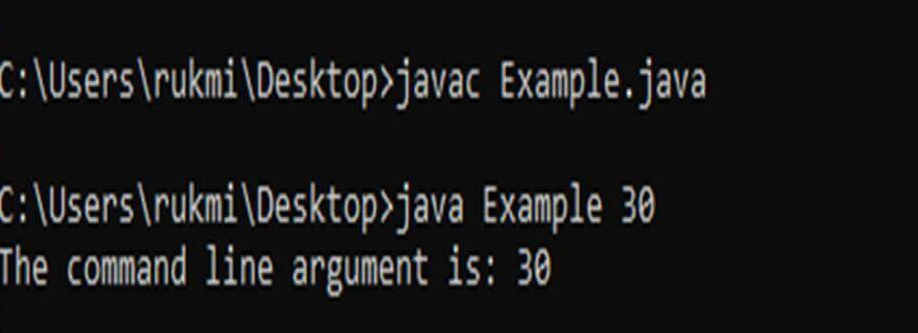Table of content

## What is an argument in Java?

An argument in java is the value passed to the variables defined in the function when the function is called. Whenever the function is called during the execution of the program, arguments are passed to the function.

An argument when passed with a function, it takes the place of those variables which are used during the function definition and the function is executed with these values.

The line below shows a function being called. Here in this example, a function sum is called. The values 20, 30 are the arguments passed to the function sum.

``total = sum(20, 30);``

After the execution of this code, the result from the sum function is stored in the variable total.

The example below illustrates how arguments can be used in a function.

``````public static int sum(int a, int b) {
return a + b; // a is replaced by and 20 and b by 30
}
public static void main(String[] args) {
int result = sum(20, 30); // 20 and 30 is passed to sum
System.out.println("Result = " + result);
}``````

The output is

``Result = 50``
The data type of the argument values passed must be the same as the parameter type defined in the function.

The arguments that are passed in the function call are called actual arguments or actual parameters. The data type of the actual parameter need not be mentioned in the function call.

The arguments can be passed in 2 ways, they are:

• Pass by value
• Pass by reference

### Pass by value

In Pass by value technique, the actual parameter's value is copied to memory and the copied values are passed to the function when the function is called.

So whatever changes made to the arguments inside the function do not reflect on the actual parameters. Because the changes are made to the copies and not to the original values.

The example program below illustrates pass by value technique

``````public class value {
public static void increment(int x, int y) {
x++;
y++;
}
public static void main(String[] args) {

int a = 10;
int b = 20;
value obj = new value(); // creating object
System.out.println("Before changing a: " + a +
" , b: " + b); // values before calling function
obj.increment(a, b); // passing arguments to function increment
System.out.println("After changing a: " +
a + " , b: " + b); // values after calling function
}
}``````

The output is

``````Before changing a: 10 , b: 20
After changing a: 10 , b: 20``````

### Pass by reference

In pass by reference technique, the reference(address) of the actual parameters are passed to the function during the function call.

Henceforth whatever changes made to the arguments inside the function are reflected on the actual parameters. The reason being when the value is changed using the reference, it changes the value of the actual variables.

The program below illustrates the pass by reference technique

``````public class reference {
static int x = 11;
static int y = 12;
public static void passbyref(reference obj) {
obj.x = 35;
obj.y = 45;
}
public static void main(String[] args) {
System.out.println("Original value of x and y : " + x + " " + y);
reference object = new reference();
passbyref(object); // passing reference of arguments to function passbyref
System.out.println("Changed value of x and y : " + x + " " + y);
}
}``````

The output is

``````Original value of x and y: 11 12
Changed value of x and y: 35 45``````

## Command-line arguments

A command-line argument is an argument that is passed along with the run command of the program. The argument passed from the console is received by the java program as input and can be used in the program.

For example, let's consider the Example program. The syntax to compile and run the program in command prompt by passing arguments is given below.

``````// Compile by
javac Example.java
//Run by
java Example 30``````They are stored as strings in the String array passed to the main function. Any number of arguments can be accepted from the console because the argument type is an array.

To access the command-line arguments inside the program, one can traverse the args array using a loop or directly use the index value of the array. The args array is the parameter passed to the main method.

Let us consider that the command line argument is 30. The program below illustrates how to take arguments from the console and access them.

``````class Example {
public static void main(String args[]) {
System.out.println("The command line argument is: " + args);
}
}``````

The output is

``The command-line argument is: 30``

The program below illustrates how to input multiple command-line arguments and access them. Let the command line arguments be Raj, 20, 80050, c.

``````class Example1 {
public static void main(String args[]) {
for (int i = 0; i < args.length; i++)
System.out.println("Command line argument" + i + " is: " + args[i]);
}
}``````

The output is

``````Command line argument0 is: Raj
Command line argument1 is: 20
Command line argument2 is: 80050
Command line argument3 is: c``````
0 results
Comment / Suggestion Section
Point our Mistakes and Post Your Suggestions